Mobile QR Code1. (School of Electrical and Computer Engineering, University of Seoul, Seoul, Korea)
2. (Samsung Electronics Inc., Hwasung, Korea)

High-speed links, clock and data recovery (CDR), adaptive equalization, continuous-time linear equalizer (CTLE), decision-feedback equalizer (DFE)

I. INTRODUCTION

As data traffic in the high-speed I/O networks has steadily increased, the demand for high-bandwidth transmission has also increased. However, the signal degradation is caused in the form of inter-symbol interference (ISI) by the bandwidth limitation in the copper-based channel as shown in Fig. 1, which gets much worse as data rates increase. To alleviate this problem, equalizer research in the high-speed I/O field has been steadily conducted, especially various continuous-time linear equalizers (CTLEs) and decision-feedback equalizers (DFEs) (1-12).

This paper proposes a receiver design that operates at data rates larger than 10 Gb/s in lossy FR4 traces with a low bit-error rates (BER) accomplished. We focus on not only improving the channel-equalization performance by adopting both an adaptive CTLE and an adaptive DFE but also reducing the area by excluding the usage of inductors such as a T-coil, a LC-VCO, and inductive peaking. Section Ⅱ describes the receiver architecture incorporating an adaptive CTLE and an adaptive DFE and suggests an offset-cancellation technique and a rectifying error-amplifier for the adaptive CTLE. Section Ⅱ also describes the DFE architecture that relaxes the timing constraint by using only a latch behind a current summer. Section Ⅲ describes the proposed adaptive 3-tap half-rate DFE and its implementation. Section Ⅲ also describes a digital low-pass filter with a hysteresis which is proposed to suppress the oscillation of the DFE tap coefficients and the related jitter. The performance measurement of the prototype chip is described in section Ⅳ. Finally, Section Ⅴ summarizes this paper.

Fig. 1. (a) Insertion loss of 6/12/18/24-inch FR4 traces and (b) single-bit pulse response in the 18-inch FR4 trace.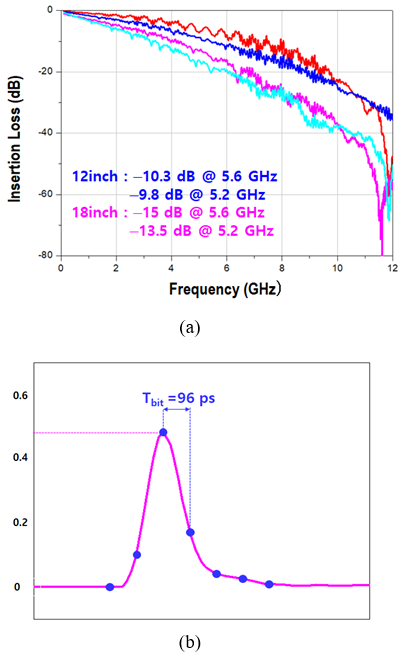Fig. 2 shows the receiver architecture. Channel equalization is performed by an adaptive CTLE and an adaptive DFE together. The equalized data is phase-detected by a clock-and-data recovery (CDR) and is deserialized by a serial-to-parallel block (S2P). The CDR is composed of a bang-bang phase detector, a charge pump, a loop filter, and a ring-VCO. To measure the receiver performance, a driver (DRV) is connected to the DFE output. The differential output of DRV is sent to an external sampling scope and a bit-error rate tester (BERT).

Fig. 3 shows the architecture of the adaptive CTLE. The high-frequency gain of the CTLE is adjusted by comparing the signals before and after a comparator (1). However, a conventional CTLE used in (1) seems to suffer from offset voltages of CLTE cells and an offset voltage between input signals (RXP and RXN). To cancel these offsets, another feedback is added to make the average of the positive CTLE output (EQP) and the average of the negative CLTE output (EQN) equal as shown in Fig. 3.

The CTLE cell is shown in Fig. 4. To perform offset cancelation, the resistance of the positive output load changes according to RCTRL. The minimum resistance is set to be about RDS || RDP and the maximum resistance is set to be RDP. To control the low-frequency gain, Rs is adjusted by a programmable control signal (EQ_DC). The output of a rectifying error-amplifier (ZCTRL) controls the capacitance of varactors ($C^{S}$) so that high-frequency gain is adaptively adjusted.

Fig. 6. Frequency responses for (a) the 18-inch FR4 trace, (b) CTLE itself, and (c) FR4 trace + CTLE (Receiver input capacitance is considered).The source-follower rectifiers used in (1) show a current consumption of about 1mA and a DC gain of about 0.88. Fig. 5 describes the rectifying error-amplifier which is an error-amplifier merged with a rectifier. This merged structure reduces the current consumption and enhances the DC gain. Stage 1 receives two pairs of differential signals from two high-pass filters located before and after the comparator. The four input transistors in Stage 1 are paired as {M1, M2} and {M3, M4}. The higher voltage of the input signals of each pair controls the output and Stage 1 plays a role of both a rectifier and an amplifier. Stage 2 amplifies the output of Stage 1 once more, generating ZCTRL.

Fig. 6 shows the simulated frequency responses for the 18-inch FR4 trace, the CTLE itself, and a conjunction of the trace and the CTLE. Considering receiver input capacitance, the channel attenuation is ${-}$15.67 dB at 5.2 GHz. And the CTLE performs high-frequency boosting to show ${-}$8.98 dB at 5.2 GHz.

CTLEs have several drawbacks. One of drawbacks is that high-frequency noise is also amplified while the high-frequency signal is boosted. Another drawback is that more than two stages of CTLEs are required for sufficient boosting. So, the bandwidth is inevitably degraded.

A DFE can complement the limited performance of a CTLE. A DFE determines whether the received data is '1' or '0' and utilizes this information to directly remove the post-cursor ISI without amplifying high-frequency noise as shown in Fig. 7.

However, the strict timing constraint for a DFE must be satisfied in order to remove the post cursor properly. Both a full-rate DFE and a half-rate DFE have the same timing constraint as follows (7,8):

(1)
$t_{CK2Q}+t_{FB}+t_{\textit{SETUP}.FF}<1UI$

In (1), $t_{CK2Q}$ stands for the clock-to-output delay of a flip-flop, $t_{SETUP.FF}$ stands for the setup time for a flip-flop, and $t_{FB}$stands for the feedback delay arising from a tap weighting and a current summer.

The timing constraint for a DFE is difficult to satisfy as a data rate increases (UI decreases). To relax the timing constraint, various methods have been proposed. One of them is replacing flip-flops next to the first flip-flop with latches (4) but the timing constraint of the first-tap feedback path is still not alleviated. Another is substituting a sample-and-hold (S/H) for a master latch in the first flip-flop (5,6). Others are merging a MUX (7) or a current summer (8) with a master latch in the first flip-flop.

To ease the burden of the timing constraint, the proposed architecture adopts the technique of using a slave latch behind a current summer but excludes other auxiliary circuits that perform a master-latch function such as a S/H (5,6), a merged latch and MUX (7), or a merged latch and current summer (8). Fig. 8 shows the illustrated block diagram of a 1-tap half-rate DFE using latches instead of flip-flops and shows the timing diagram. In Fig. 8(b), $t_{SETUP.LAT}$ stands for the time required for $De_{n}$ to be stored reliably by ‘Low’ of DCKB, $t_{DQ.LAT}$ stands for the input-to-output delay of a latch, and $t_{FB}$ stands for the feedback delay. The timing constraint in Fig. 8(a) is written as follows:

Fig. 8. Illustrated (a) block diagram of 1-tap half-rate DFE using latches and (b) timing diagram.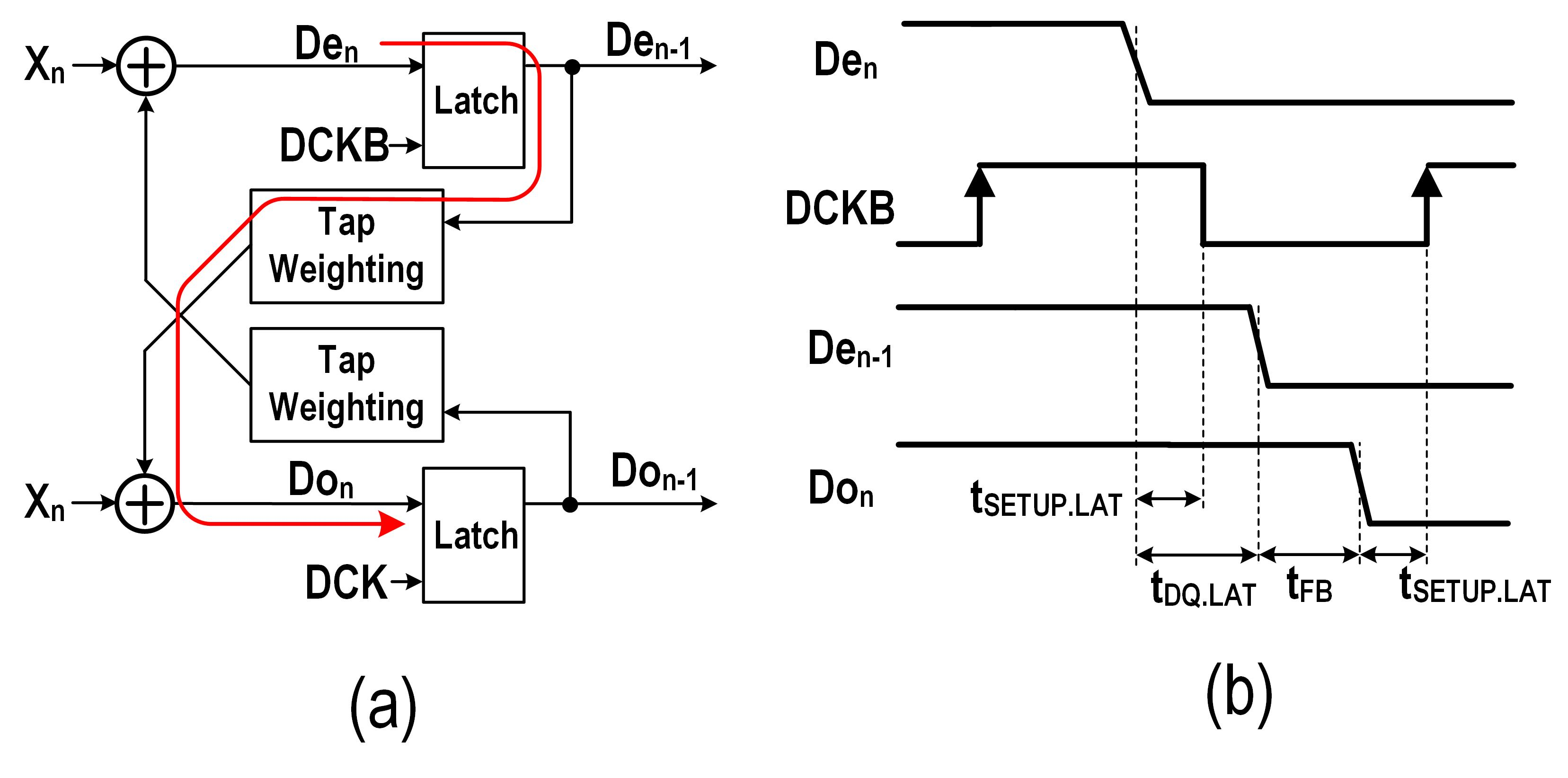(2)
\begin{equation*} \left(t_{DQ.LAT}- t_{\textit{SETUP}.LAT}\right)+t_{FB}+t_{\textit{SETUP}.LAT} \end{equation*} $=~ t_{DQ.LAT}+t_{FB}<1UI$

According to simulation results, $t_{DQ.LAT}$ is about 40 ps while the minimum shifting delay of a flip-flop, $t_{SHIFT.FF} $=t_{CK2Q}+t_{SETUP.FF}$$, is about 85 ps. Here, the minimum shifting delay of a flip-flop means the minimum input-to-output delay of a flip-flop (13). Since is measured about 10 ps, the left side of (1) is about 95 ps while that of (2) is about 50 ps. Thus, the timing constraint can be greatly mitigated by using only a latch after a current summer as shown in Fig. 8(a).

Due to the transparent nature of a latch, two issues need to be considered: the data racing and the recovered clocks’ misalignment. There is no data-racing problem because each of the two latches of Fig. 8(a) operates in either holding or sensing mode, respectively, with the opposite clock phases. The recovered clocks’ misalignment issue is addressed in the following section.

Fig. 9 shows the proposed architecture of a 3-tap half-rate DFE. This structure is composed of the even-data path, the even-edge path, the odd-data path, and the odd-edge path. Both the even-data and odd-data paths use only latches instead of flip-flops to relax the timing constraint. However, both the even-edge and odd-edge paths still rely on the first flip-flops for optimal data-edge detection, which means that the edge clocks (XCK and XCKB) track data’s transition edges correctly. A current summer has a conventional structure (3) and a latch has a current-mode logic (CML) type. For the even data, the post cursors are removed by Do$_{n-1}$, $De_{n}$$_{n-2}, and Do_{n-3}, corresponding to data before 1 UI, 2 UI, and 3 UI, respectively. For even edge, the post cursors are removed by Do_{n-2}, Do_{n-3}, and Do_{n-4}. Fig. 10 shows an illustrated timing diagram of Fig. 9. For instance, the post cursors in the even data ‘G_{T_{8}}’ at T_{8} are removed by ‘FT_{8}’, ‘ET_{8}’, and ‘DT_{8}’ at T_{8}, which correspond to Do_{n}$$_{n-1}$, De$_{n-2}$, and Do$_{n-3}$, respectively. The post cursors in the even edge ‘GT9’ at T9 are removed by ‘F$_{T9}$’, ‘E$_{T9}$’, and ‘D$_{T9}$’ at T9.

Fig 11. (a) Architecture of sign-sign LMS, examples of sign-sign LMS algorithm when (b) ${\textit{E}}$$_{n} {\textless} 0 and (c) {\textit{E}}$$_{n}$ {\textgreater} 0, and (d) implementation architecture for a half-rate DFE.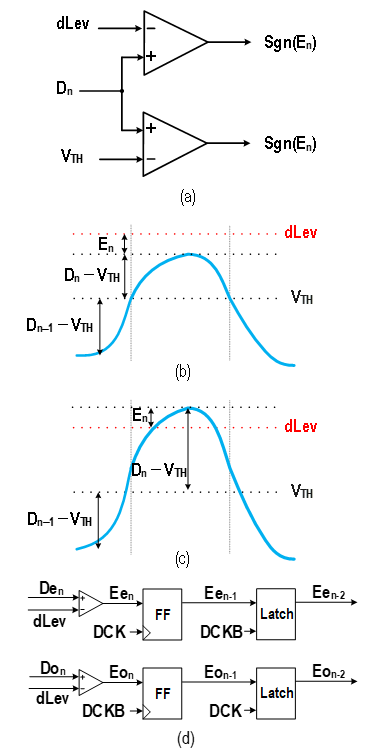Fig. 12. Block diagram of the adaptive DFE for the 1$^{\mathrm{st}}$ tap and ${\textit{dLev}}$.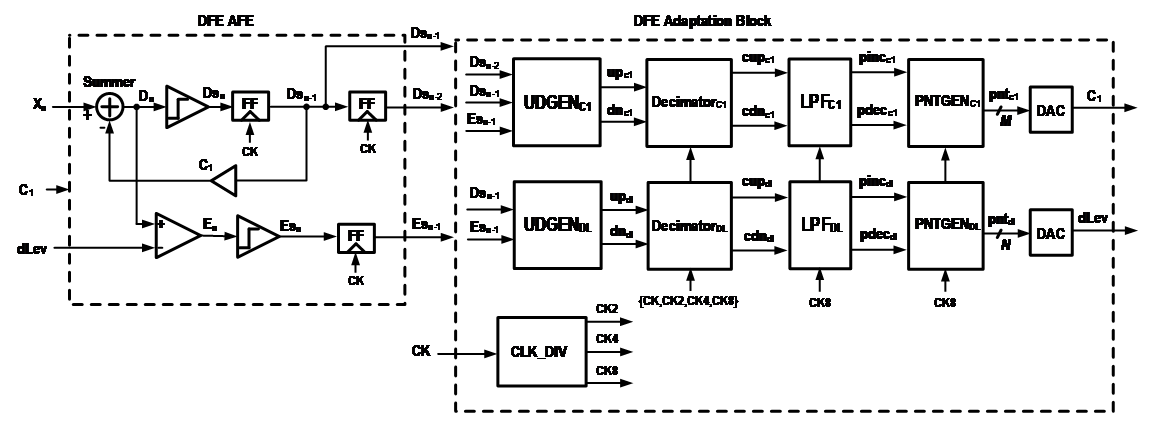1. Adaptation Algorithm: Sign-Sign Least Mean Square

Sign-sign least mean square (sign-sign LMS) algorithm is well known as one of the easiest ways to implement DFE adaptation in a circuit level, requiring only the sign of a current error and the sign of the previous data as shown in Fig. 11(a) (14,2).

Fig. 11(b) and (c) shows an example of the adaptation algorithm when D$_{n-1}$ < 0 and D$_n$ > 0, that is sgn(D$_{n-1}$) = ${-}$1 and sgn(D$_n$) = +1. $D_{n}$ stands for the output of the summer and $E_{n}$ stands for the error of $D_{n}$ with respect to the desired level, dLev ( ).

In the case of $E_{n}$ < 0 as shown in Fig. 11(b), $C_{1}$ increases by 1 and dLev decreases by μ$_{dLev}$ according to (3) and (4). Conversely, in the case of $E_{n}$ > 0 as shown in Fig. 11(c), $C_{1}$ decreases and dLev increases. Regardless of $E_{n}$, $C_{1}$ and dLev are adjusted in such a way that the difference between $D_{n}$ and dLev at the sampling instant becomes the minimum.

(3)
$C_j [n+1]=C_j [n]+μ_j sgn⁡(D_{n-j} ) sgn⁡(E_n )ifD_n>0$

(4)
$dLev[n+1]=dLev[n]+μ_{dLev} sgn⁡(E_n )ifD_n>0$

Fig. 11(d) shows the implementation architecture of sign-sign LMS for the half-rate DFE.

2. Digital Low-pass Filter with a Hysteresis

Fig. 12 illustrates the block diagram of the analog front-end (DFE AFE) and the adaptation block. Although the actual design is an adaptive 3-tap half-rate DFE with partially latches and flip-flops, a 1-tap full-rate DFE with only flip-flops is used instead for the simplified explanation. $E_{n}$ is generated by comparing the summer output ($D_{n}$) to dLev. As $E_{n}$ passes through a slicer, the sign value of $E_{n}$ ($Es_n$) is generated. $Es_n$ has a value of +1 or ${-}$1. When $Es_n$ is sampled by the rising edge of CK, Es$_{n-1}$ is generated. These output signals of DFE AFE block are used as inputs for the UP/DOWN generator for $C_{1}$ ($UDGEN_{$C_{1}$}$) and dLev ($UDGEN_{DL}$).

Fig. 12. Block diagram of the adaptive DFE for the 1$^{\mathrm{st}}$ tap and ${\textit{dLev}}$.Fig. 13. Block diagrams of (a) hysteresis LPF and (b) INCDEC. (c) Hysteresis increase/decrease of ${\textit{pcnt}}$.Flip-flops are used to mitigate the timing burden of DFE AFE block. If the same number of clock cycles (i.e., the same delay times) are maintained between the entire set of the signals, $UDGEN_{$C_{1}$}$ and $UDGEN_{DL}$ can operate properly.

Fig. 13(a) shows the block diagram of the low-pass filter ($LPF_{C1}$) for $C_{1}$ shown in Fig. 12. The LPF receives UP/DOWN signals from UDGEN block and performs low-pass filtering by using an internal counter. Fig. 13(b) shows the configuration of INCDEC block in Fig. 13(a). It is assumed that the data has 8 bits, but it can have any number of bits. Since ncnt[7:0] and pcnt[7:0] are two's compliment data, MSBs stand for signed values.

INCDEC block receives cup/cdn and pcnt(7) as inputs in order to determine the increment or the decrement step (idstep). According to idstep determined, pcnt either increases or decreases as shown in Fig. 13(c). The method of determining idstep is as follows.

(a) In the case of {cup, cdn} = {0,0}, idstep = 0.

(b) In the case of {cup, cdn} = {0,1}, idstep = ${-}$1 or ${-}$3 depending on pcnt(7) = 1 or 0, respectively.

(c) In the case of {cup, cdn} = {1,0}, idstep = +3 or +1 depending on pcnt(7) = 1 or 0, respectively.

The two different step sizes are specified for the increment step or the decrement step. For example, if cdn = 1 when pcnt is positive (i.e., pcnt(7) = 0), pcnt changes by ${-}$3, which makes itself closer to 0. Conversely, if cup = 1 when pcnt is negative (i.e., pcnt(7) = 1), pcnt changes by +3, which makes itself closer to 0. This method inhibits the outputs of the LPF from being activated, thereby suppressing the oscillation of the DFE tap coefficients and dLev.

Of course, the increment/decrement step can have any ratio (e.g., +1/+1 or +4/+1). However, if the ratio becomes too small, it may cause the DFE tap coefficients and dLev to oscillate. On the contrary, if the ratio becomes too large, the DFE tap coefficients and dLev may not converge to the optimum value. In this paper, the increment/decrement step is determined to have a ratio of 3 (i.e., +3/+1 and ${-}$3/${-}$1). As shown in Fig. 13(b), a signal Add_lsb is generated and connected to the second full-adder to realize this ratio of 3.

A low-pass filter for dLev ($LPF_{DL}$) has the same structure as $LPF_{C1}$. However, by making the bit numbers of the counters in $LPF_{C1}$ and $LPF_{DL}$ different, we solve the stability issue that may occur when two feedback loops operate simultaneously.

3. DFE Adaptation Block and Verilog Simulation

pinc signal is asserted when pcnt[7:6] reaches '01' and pdec signal is asserted when pcnt[7:6] reaches '10'. In Fig. 12, the outputs of $LPF_{C1}$ ($pinc_{c1}$ and $pdec_{c1}$) control the pointer-generation block (PNTGENC1) for $C_{1}$. If $pinc_{c1}$ = 1, the output signal of PNTGENC1, pntc1, increases by +1 until it reaches the maximum value. Conversely, if $pdec_{c1}$ = 1, pntc1 decreases by ${-}$1 until it reaches the minimum value. Similarly, the outputs of $LPF_{DL}$ ($pinc_{dl}$ and $pdec_{dl}$) control PNTGENDL for dLev. PNTGENC1 and PNTGENDL have the same configuration but the bit numbers may be different. pntc1 and $pnt_{dl}$ are converted to analog signals ($C_{1}$ and dLev), respectively, through DACs. $C_{1}$ and dLev are fed back to DFE AFE block.

Fig. 14. Verilog simulation results: (a) DFE coefficients without hysteresis low-pass filter and (b) with hysteresis low-pass filter. (c) Relationship between summer outputs and sampling clocks.Fig. 14 shows Verilog simulation results of the receiver. Verilog modeling includes a lossy channel, a CDR, and an adaptive 3-tap half-rate DFE as shown in Fig. 9. The CDR updates the output-clock phase at every clock cycle (15). Since Verilog modeling can enable the behavioral simulation of the receiver system, it can significantly reduce the simulation time for the design of the entire DFE architecture and each building block compared to the SPICE simulation.

Using a digital low-pass filter without a hysteresis as shown in Fig. 14(a), each DFE tap coefficient ($C_{1}$, $C_{2}$, $C_{3}$) and dLev show periodic oscillation by 3 to 4 steps in the steady state. This oscillation may cause additive noise on the output of summer, $D_{n}$, distorting data transitions and increasing jitter and BER.

On the other hand, when using the digital low-pass filters with a hysteresis as shown in Fig. 14(b), there is no oscillation in the tap DFE coefficients and dLev. Of course, the final convergence values are the same as those in the case of Fig. 14(a). Thus, the perturbation of $D_{n}$ and the jitter can be greatly suppressed.

Fig. 15. Pictures of (a) RX layout and (b) die micro photograph.Fig. 14(c) shows that the rising edges of the data sampling clock (DCK or DCKB) coincide with the midpoint of each data bit of the summer output ($De_{n}$ or $Do_{n}$).

IV. MEASUREMENT

The receiver test chip was designed and fabricated in 28-nm CMOS process. The RX layout and a die microphotograph are shown in Fig. 15. The active area of the chip is 980600 μm$^{2}$, where the adaptive DFE occupies 32080 μm$^{2}$ and the adaptive CTLE occupies 230100 μm$^{2}$.

Fig. 16 shows the test environment for measuring jitter, eye diagrams, and the BER of the data. A BERT provides a trigger clock for a sampling scope and a reference clock for a device under test (DUT) and generates PRBS patterns. ISI Board provides various lengths of FR4 traces. The BERT-generated PRBS pattern passes through ISI Board and becomes the input of the DUT. The data recovered by the DUT is analyzed by the sampling scope and the BERT.

Unless otherwise stated, all measurements are conducted with both the adaptive CTLE and adaptive DFE activated. PRBS 2$^7$ patterns are used and pre-emphasis is not applied during the measurements. The 1-V supply is used.

When using the 18-inch FR4 trace, no bit error was detected while transferring more than 10$^{14}$ data at 10.4 Gb/s but the BER increased as the data rate became larger than 10.4 Gb/s. When using the 12-inch FR4 trace, no bit error was detected while transferring more than 10$^{14} data at 11.2 Gb/s but the BER increased as the data rate became larger than 11.2 Gb/s. Fig. 17. Eye diagram and jitter histogram of (a) channel output and (b) recovered data when data rate is 10.4 Gb/s.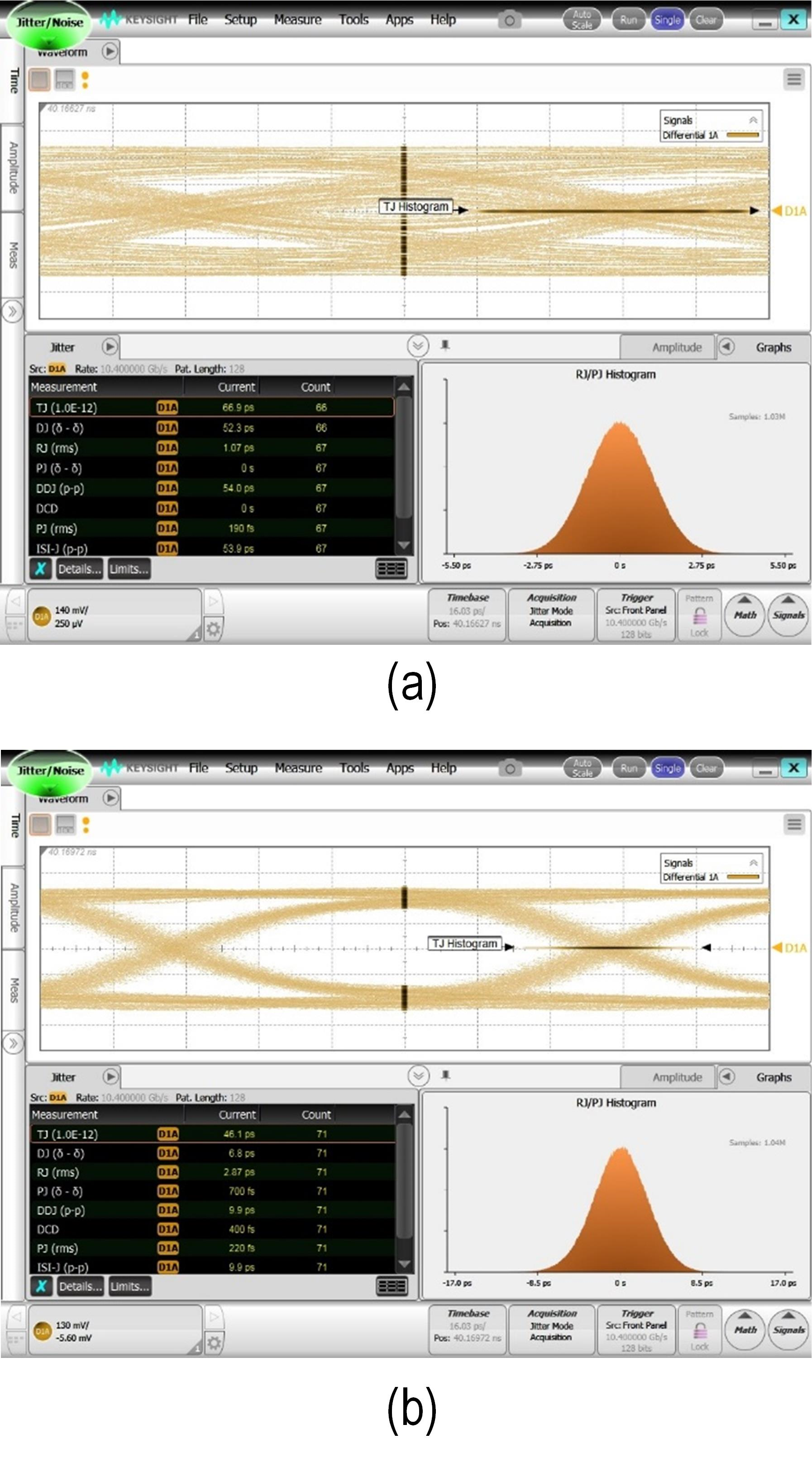Fig. 17(a) shows the measured eye diagram and jitter histogram at 10.4 Gb/s after the 18-inch FR4 trace. The measured jitter is as follows. DJ (deterministic Jitter) = 52.3 ps, RJ (random Jitter) = 1.07 ps, and TJ (total Jitter) = 66.9 ps. The measurement results show that ISI accounts for most of the DJ. Fig. 17(b) shows the measured eye diagram and jitter histogram of recovered data, which is the output of the driver. Since the driver is driven by recovered clocks, it contains all the jitter components of the clocks. Of course, due to the jitter of the driver itself, the final jitter of the driver is expected to become larger. The measured jitter is as follows. DJ = 6.8 ps, RJ = 2.87 ps, and TJ = 46.1 ps. The operation of the equalizer and CDR reduces DJ by 45.5 ps but increases RJ by 1.8 ps. Fig. 18(a) shows the measured eye diagram and jitter histogram at 11.2 Gb/s after the 12-inch FR4 trace. The measured jitter is as follows. DJ = 26.4 ps, RJ = 1.08 ps, and TJ = 41.1 ps. Fig. 18(b) shows the measured eye diagram and jitter histogram of recovered data. The measured jitter is as follows. DJ = 10.5 ps, RJ = 2.53 ps, and TJ = 45.1 ps. Fig. 18. Eye diagram and jitter histogram of (a) channel output and (b) recovered data when data rate is 11.2 Gb/s.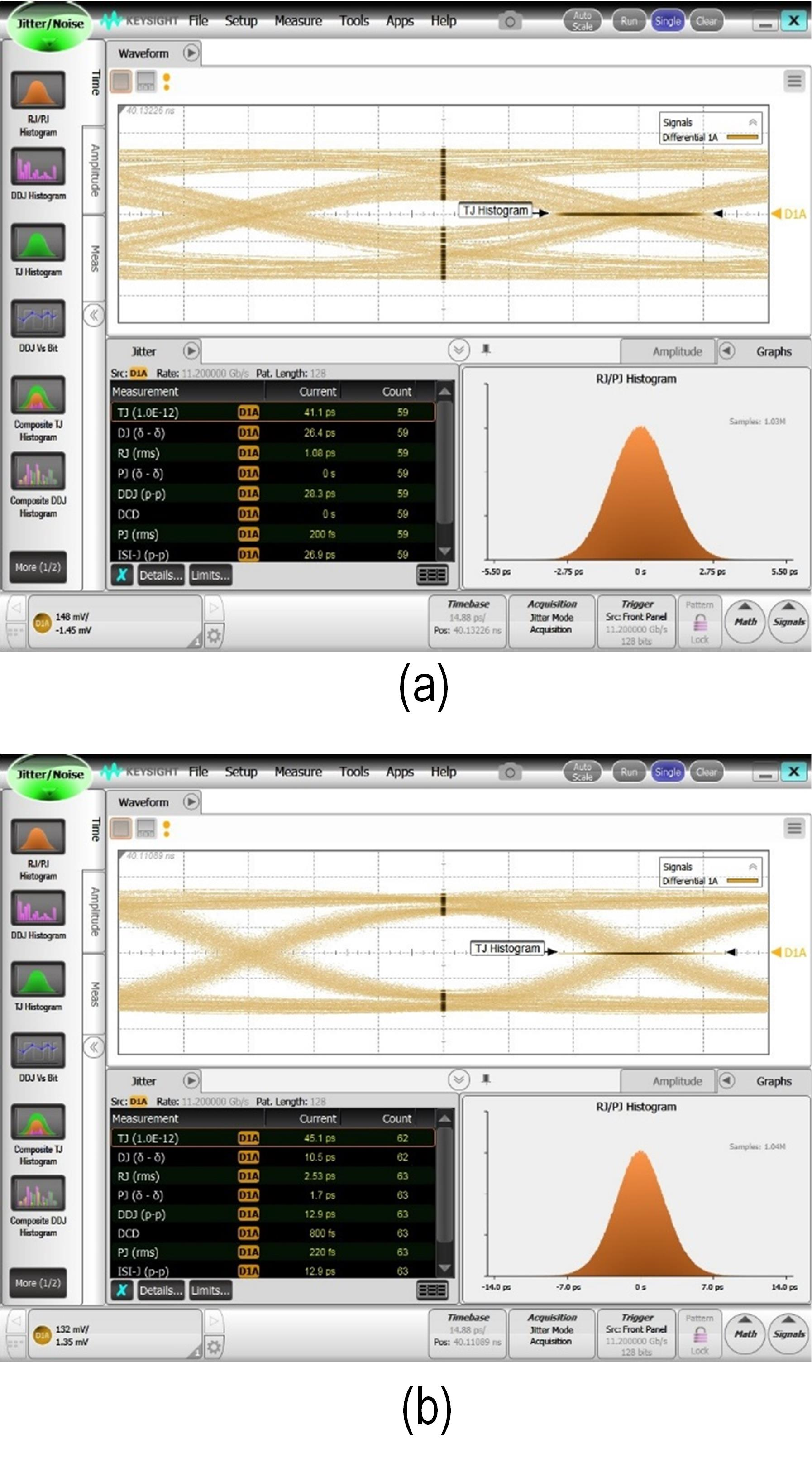Fig. 19. Jitter tolerance curves for (a) recovered 10.4-Gb/s data and (b) recovered 11.2-Gb/s data.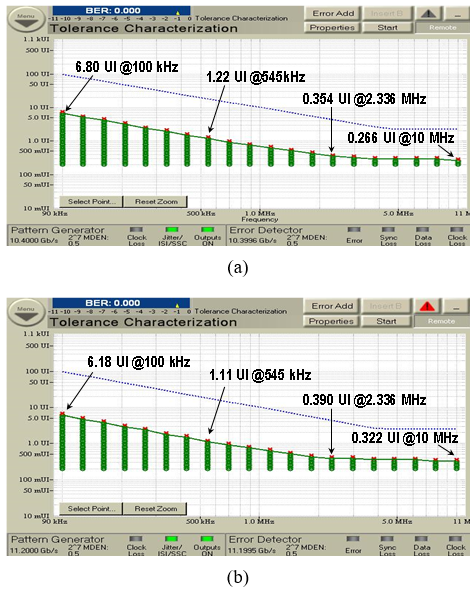Fig. 19 shows the measured jitter tolerance curves at 10.4-Gb/s and 11.2-Gb/s, respectively. The receiver has jitter tolerance of 0.266 UI and 0.322 UI at 10 MHz, respectively, with tracking bandwidths of about 2 MHz. Table 1. Performance Comparison Fig. 20 shows the total power breakdown of the receiver measured at 11.2 Gb/s. The measured power consumption excluding the driver is 60 mW and the energy efficiency is calculated as 5.36 pJ/bit. Table 1 summarizes the results of this receiver in comparison with other receivers having both a CTLE and a DFE. This receiver and the ones in (9,12) perform both CTLE and DFE adaptation. Compared to other receivers in (9-12), this receiver has a relatively very small BER (<$10^{-14}$). V. CONCLUSIONS This paper has described a receiver design that incorporates both an adaptive CTLE and an adaptive DFE. In the proposed CTLE, a merged rectifying error-amplifier reduces the current consumption and enhances the DC gain. Offset cancelation for the CTLE is performed by adaptively adjusting the load resistance of a CTLE cell. The proposed adaptive DFE relaxes the timing constraint using only a CML latch behind a current summer without other auxiliary circuits that perform a master-latch function. The DFE suppresses the oscillation of the DFE tap coefficients and the data level in the steady state utilizing a digital low-pass filter with a hysteresis. The receiver is fabricated in 28-nm CMOS process and occupies 980600 μm$^{2}$. The measured BER is less than$10^{-14}\$ at 10.4 Gb/s for an 18-inch FR4 trace and at 11.2 Gb/s for a 12-inch FR4 trace, respectively, with both the adaptive CTLE and the adaptive DFE activated. In 11.2 Gb/s, the energy efficiency of the receiver is 5.36 pJ/bit.

ACKNOWLEDGMENTS

This research was supported by the MOTIE (Ministry of Trade, Industry & Energy (10080285) and KSRC (Korea Semiconductor Research Consortium) support program for the development of the future semiconductor device.

This paper is supported by Future Interconnect Technology Cluster Program of Samsung Electronics.

The EDA Tool was supported by the IC Design Education Center.

REFERENCES

1
Choi J.-S., Hwang M.-S., Jeong D.-K., Mar 2004, A 0.18-m CMOS 3.5-Gb/s Continuous-Time Adaptive Cable Equalizer Using Enhanced Low-Frequency Gain Control Method, IEEE J. Solid-State Circuits, Vol. 39, No. 3, pp. 419-4252
Stojanovic V., Ho A., Garlepp B. W., Chen F., Wei J., Tsang G., Alon E., Kollipara R. T., Werner C. W., Zerbe J. L., Horowitz M. A., Apr 2005, Autonomous Dual-Mode (PAM2/4) Serial Link Transceiver with Adaptive Equalization and Data Recovery, IEEE J. Solid-State Circuits, Vol. 40, No. 4, pp. 1012-10263
Beukema T., Sorna M., Selander K., Zier S., Ji B. L., Murfet P., Mason J., Rhee W., Ainspan H., Parker B., Beakes M., Dec 2005, A 6.4-Gb/s CMOS SerDes Core with feed-forward and decision-feedback equalization, IEEE J. Solid-State Circuits, Vol. 40, No. 12, pp. 2633-26454
Emami-Neyestanak A., Varzaghani A., Bulzacchelli J. F., Rylyakov A., Ken Yang C.-K., Friedman D. J., Apr 2007, A 6.0-mW 10.0-Gb/s Receiver with Switched-Capacitor Summation DFE, IEEE J. Solid-State Circuits, Vol. 42, No. 4, pp. 889-8965
Wong K.-L., Rylyakov A., Yang C.-K. K., Apr 2007, A 5-mW 6-Gb/s Quarter-Rate Sampling Receiver With a 2-Tap DFE Using Soft Decisions, IEEE J. Solid-State Circuits, Vol. 42, No. 4, pp. 881-8886
Rylyakov A., Jun 2007, An 11 Gb/s 2.4 mW Half-Rate Sampling 2-Tap DFE Receiver in 6Snm CMOS, in Symp. VLSI Circuits Dig. Tech. Papers, pp. 272-2737
Ibrahim S., Razavi B., Jun 2011, Low-Power CMOS Equalizer Design for 20-Gb/s Systems, IEEE J. Solid-State Circuits, Vol. 46, No. 6, pp. 1321-13368
Lu Y., Alon E., Dec 2013, Design Techniques for a 66 Gb/s 46 mW 3-Tap Decision Feedback Equalizer in 65 nm CMOS, IEEE J. Solid-State Circuits, Vol. 48, No. 12, pp. 3243-32579
Parikh Samir, Kao Tony, Hidaka Yasuo, Jiang Jian, Toda Asako, Mcleod Scott, Walker William, Koyanagi Yochi, Shibuya Toshiyuki, Yamada Jun, Feb 2013, A 32Gb/s Wireline Receiver with a Low-Frequency Equalizer, CTLE and 2-Tap DFE in 28nm CMOS, in IEEE Int. Solid-State Circuits Conf. Dig. Tech. Papers, pp. 28-2910
Dickson T. O., Liu Y., Rylov S. V., Agrawal A., Kim S., Hsieh P. H., Bulzacchelli J. F., Ferriss M., Ainspan H. A., Rylyakov A., Parker B. D., Beakes M. P., Baks C., Shan L., Kwark Y., Tierno J. A., Friedman D. J., Aug 2015, A 1.4 pJ/bit, Power-Scalable 16×12 Gb/s Source-Synchronous I/O With DFE Receiver in 32 nm SOI CMOS Technology, IEEE J. Solid-State Circuits, Vol. 50, No. 8, pp. 1979-193111
Han J., Sutardja N., Lu Y., Alon E., Dec 2017, Design Techniques for a 60-Gb/s 288-mW NRZ Transceiver With Adaptive Equalization and Baud-Rate Clock and Data Recovery in 65-nm CMOS Technology, IEEE J. Solid-State Circuits, Vol. 52, No. 12, pp. 3474-348512
Park K., Lee J., Lee K., Choo M.-S., Jang S., Chu S.-H., Kim S., Jeong D.-K., Exp Briefs, A 55.1 mW 1.62-to-8.1 Gb/s Video Interface Receiver Generating up to 680 MHz Stream Clock Over 20 dB Loss Channel, IEEE Trans. Circuits Syst. II13
Moon Y., Cho Y.-H., Lee H.-B., Jeong B.-H., Hyun S.-H., Kim B.-C., Jeong I.-C., Seo S.-Y., Shin J.-H., Choi S.-W., Song H.-S., Choi J.-H., Kyung K.-H., Jun Y.-H., Kim K., 2009, 1.2V 1.6Gb/s 56nm 6F2 4Gb DDR3 SDRAM with Hybrid-I/O Sense Amplifier and Segmented SubArray Architecture, in IEEE Int. Solid-State Circuits Conf. Dig. Tech. Papers, pp. 128-13014
Widrow B., McCool J. M., Larimore M. G., Johnson Jr. C. R., Aug 2005, Stationary and Nonstationary Learning Characteristics of the LMS Adaptive Filter, in Proc. IEEE, Vol. 64, No. 8, pp. 1151-116215
Gardner F. M., Nov 1980, Charge-Pump Phase-Locked Loops, IEEE Trans. Communications, Vol. COM-28, pp. 1849-1858Author

Young-Gil Go was born in Sokcho, Korea, in 1994.

He received the B.S. and the M. S. degrees in the School of Electrical and Computer Engi-neering, University of Seoul, Seoul, Korea, in 2019 and 2021.

In 2021, he joined Samsung Electronics, Hwa-sung, Korea.

His research interests include clock and data recovery for high-speed communication and high-speed I/O interface circuits.

Hye-Seong Shin was born in Incheon, Korea, in 1993.

He received the B.S. and the M. S. degrees in the School of Electrical and Computer Engineering, University of Seoul, Seoul, Korea, in 2019 and 2021.

In 2021, he joined Samsung Electronics, Hwasung, Korea.

His current research interests include clock and data recovery for high-speed communication and high-speed I/O interface circuits.

Jae-Geol Lee was born in Seoul, Korea, in 1995.

He received the B.S. degree in the School of Electrical and Computer Engineering, University of Seoul, Seoul, Korea, in 2020.

He is currently working toward the M. S. degree at the same university.

His research interests include PAM4 Signaling for high-speed communication and high-speed I/O interface circuits.

Hyun-Woo Ahn was born in Changwon, Korea, in 1994.

He received the B.S. degrees in the Physics and Electronic Physics, University of Seoul, Seoul, Korea, in 2020.

He is currently working toward the M. S. degree in the Electrical and Computer Engineering at the same university.

His research interests include PAM4 Signaling for high-speed communication and high-speed I/O interface circuits.

Yo-Han Kim was born in Daejeon, Korea, in 1995.

He received the B. S. degree in the School of Electrical and Computer Engineering, University of Seoul, Seoul, Korea, in 2020.

He is currently working toward the M.S. degree at the same university.

His current research interests include PAM4 Signaling for high-speed communication and high-speed I/O interface circuits.

Hyeon-Jin Yang was born in Yecheon, Korea, in 1995.

He received the B. S. and the M. S. degrees in the School of Electrical and Computer Engineering, Univer-sity of Seoul, Seoul, Korea, in 2018 and 2020, respectively.

His current research interests include CDR for high-speed communication and high-speed I/O interface circuits.

Myung-Hun Jung was born in Gyeonggi-do, Korea, in 1990.

He received the B. S. and the M. S. degrees in the School of Electrical and Computer Engineering, Univer-sity of Seoul, Seoul, Korea, in 2018 and 2020, respectively.

In 2020, he joined Samsung Electronics, Hwasung, Korea.

His current research interests include PLL and LC-VCO circuits for high-speed communication and high-speed I/O interface circuits.

Yongsam Moon (S’96-M’01) received the B.S., M.S., and Ph.D. degrees in electronics engineering from Seoul National University, Seoul, Korea, in 1994, 1996, and 2001, respectively.

From 2001 to 2002, he was with Inter-University Semiconductor Research Center in the same university as a research engineer.

In 2002, he joined Silicon Image Inc, Sunnyvale, CA, where he developed various high-speed serial links as a Member of Technical Staff.

In 2006, he joined Samsung Electronics, Hwasung, Korea, where he was involved in the design of DRAM products.

In 2009, he joined the faculty of the School of Electrical and Computer Engineering, University of Seoul, Seoul, Korea.

He is currently a Professor.

His current research interests include clock and data recovery for high-speed communication and high-speed I/O interface circuits.